Mechanical Engineering - Mechanics                                 Home : www.sharetechnote.com Mechanics : Centroid 2D   Centroid of a figure is the center of gravity when the weight is uniformly distributed throughout the figure. In a mathematical context, centroid of a figure is the average position of all points in a given shape. One can draw all the connecting lines to the centroid to all the vertices of the figure and when all the connecting lines are equal in length, then the point that connects all vertices is the centroid of the figure. When the density of the figure is constant, the weight of the figure is uniformly distributed. If the density of the figure is not constant, the location of centroid and center of gravity is different, as center of gravity is mostly affected by the weight distribution of an object.   The first year Mechanics course for engineering students involve locating the centroid of figures given as f(x) with integral calculus. All the figures will have uniform density, indicating uniform weight distribution. Location of the centroid is where the weight always acts through in an object and the location is used for both static and dynamic rigid body equilibrium analysis later on in the mechanics course and upper year mechanics course.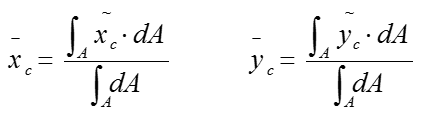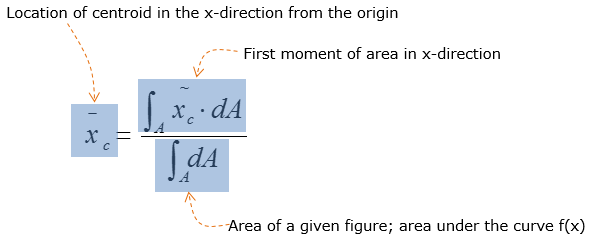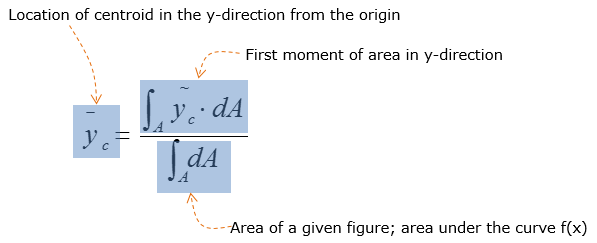To get a good feel for calculating the location of centroids using integral calculus, there are easy, moderate, and hard example questions that are prepared for you One of the 3 types of questions would most likely show up on the midterm or exam for the 1st year mechanics course for engineering students     Example 01 >   Consider the given figure below: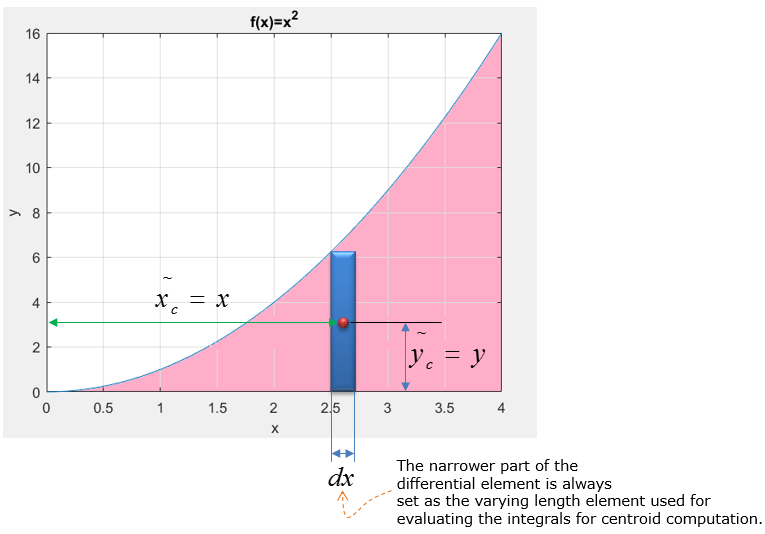Now that the arbitrary location of the centroid in the x and y direction are computed using the differential element, calculate for the location of centroid in the x and y direction.   For any centroid calculations, start off by calculating the shaded area bounded under a given curve as it is easier to get part marks.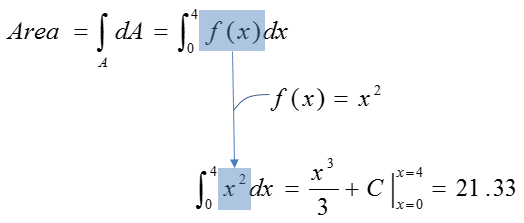Since the area is known, evaluate the integral for the first area of moment for both x and y locations.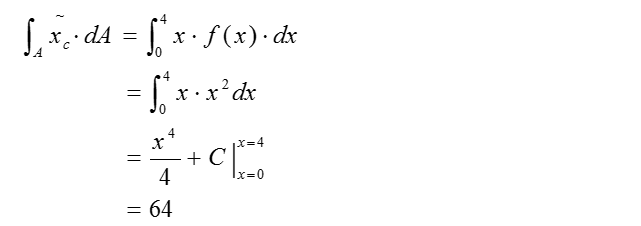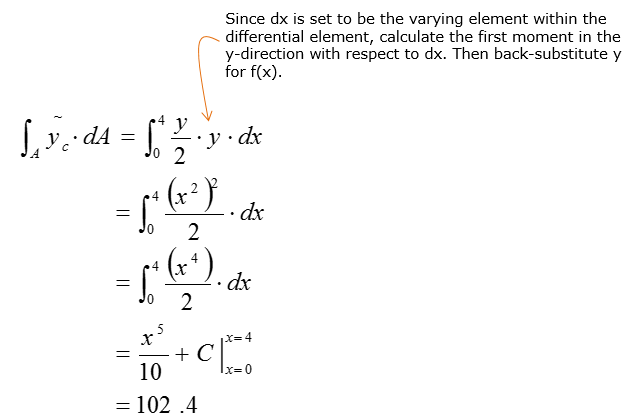Divide the first moment of area by area of the shaded figure to compute for the location of centroid in the x and y directions.   Consider the x-direction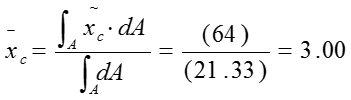Consider the y-direction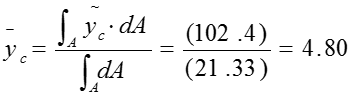Therefore the centroid is located 3.00 units to the right and 4.80 units above the origin